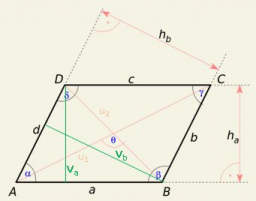# Rhombus construct

Construct parallelogram (rhombus) ABCD, | AB | = 4 cm alpha = 30° and | BD | = 5 cm.

n =  0Did you find an error or inaccuracy? Feel free to write us. Thank you!

Showing 1 comment:
Dr Math
approach:
1. draw useck AB
2 angle ABX = alpha = 30 °
2. circle k1 (B; r = | BD | = 5 cm)
3. D is the intersection of the angle arm BX and k1
4 draw the parallel DY with the side AB
5. circle k2 (D; r = | AB | = 4 cm)
6. C is the intersection of k2 and the line DY
7. connect the ABCD points to the rhombus

#### Grade of the word problem:

We encourage you to watch this tutorial video on this math problem: